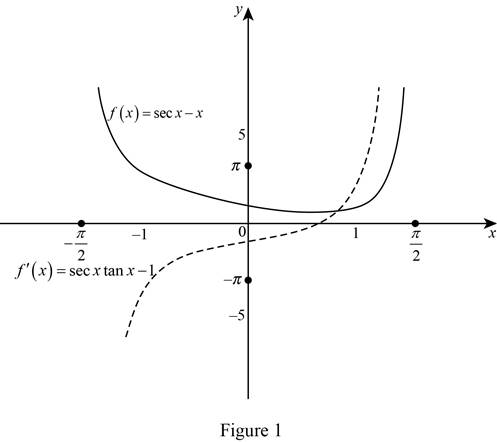# The derivative of the function f ( x ) = sec x − x .### Single Variable Calculus: Concepts...

4th Edition
James Stewart
Publisher: Cengage Learning
ISBN: 9781337687805### Single Variable Calculus: Concepts...

4th Edition
James Stewart
Publisher: Cengage Learning
ISBN: 9781337687805

#### Solutions

Chapter 3.3, Problem 25E

(a)

To determine

## To find: The derivative of the function f(x)=secx−x.

Expert Solution

The derivative of f(x) is f(x)=secxtanx1_.

### Explanation of Solution

Given:

The function is f(x)=secxx.

Derivative rules:

(1) Difference Rule: ddx[f(x)g(x)]=ddx[f(x)]ddx[g(x)]

(2) Power Rule: ddx(xn)=nxn1

Calculation:

Obtain the derivative of f(x).

f(x)=ddx(f(x)) =ddx(secxx)

Apply the difference rule (1),

f(x)=ddx[secx]ddx[x]=secxtanxddx[x]

Apply the power rule (2) and simplify further,

f(x)=secxtanx[1x11]=secxtanx1

Therefore, the derivative of f(x) is f(x)=secxtanx1_.

(b)

To determine

### To check: The derivatives of f(x) obtained in part (a) is reasonable or not by using the graphs of f(x) and f′(x).

Expert Solution

The derivatives of f(x) is reasonable.

### Explanation of Solution

Graph:

Use the online graphing calculator and draw the graph of f(x)=secxx and f(x)=secxtanx1 as shown below in Figure 1.Observation:

From Figure 1, it is noticed that,

If f(x) is positive then f(x) is increasing function.

If f(x) is negative then f(x) is decreasing function.

If f(x) crosses the x axis (f(x)=0), then f(x) is local extrema (that is, local minimum or local maximum).

Therefore, it can be concluded that the derivative of the function f(x)=secxx is reasonable.

### Have a homework question?

Subscribe to bartleby learn! Ask subject matter experts 30 homework questions each month. Plus, you’ll have access to millions of step-by-step textbook answers!